# Fraction Equivalence and Ordering

## Objective

Recognize and generate equivalent fractions with smaller units using tape diagrams.

## Common Core Standards

### Core Standards

?

• 4.NF.A.1 — Explain why a fraction a/b is equivalent to a fraction (n × a)/(n × b) by using visual fraction models, with attention to how the number and size of the parts differ even though the two fractions themselves are the same size. Use this principle to recognize and generate equivalent fractions.

?

• 3.NF.A.3

• 4.OA.A.1

• 4.OA.A.2

## Criteria for Success

?

1. Understand that equivalent fractions are fractions that refer to the same whole and are the same size.
2. Generate equivalent fractions with smaller units using visual models, namely tape diagrams (MP.5).
3. Show that two fractions are equivalent using visual models, and explain why the models demonstrate their equivalence (MP.3).
4. Understand that equivalence is valid only when two fractions refer to the same whole.

## Tips for Teachers

?

• Students should be familiar with equivalent fractions and how to recognize and generate simple ones from Grade 3 (3.NF.3).
• Depending on students’ comfort level with key representations for fractions from Grade 3, particularly tape diagrams/fraction strips and number lines, Lessons 1 and 2 can be combined.
• The standards state that work with fractions in Grade 4 is limited to fractions with denominators 2, 3, 4, 5, 6, 8, 10, 12, and 100. However, on his blog Mathematical Musings, Bill McCallum notes that “The restrictions in the standards are intended to both restrict what can be put on an assessment, and to put the brakes on over-enthusiastic focus on one part of the standards; they aren’t intended to forbid the use of examples that time and common sense allow” (General questions about the Mathematics standards, Mathematical Musings). Thus, in this lesson and throughout the unit, students are exposed to and asked to work with fractions whose denominators may not be included in that list, with the intention of not limiting their understanding.
• As a supplement to the Problem Set, students can play a game to practice finding equivalent fractions with smaller units using tape diagrams, such as “Show Me 2” from Games with Fraction Strips and Fraction Cards on The Max Ray Blog.
• There are no problems in this Problem Set, or any hereafter, that are related to the idea that “comparisons are valid only when the two fractions refer to the same whole” (4.NF.2). (Note that the idea of equivalence is a type of comparison.) This is because this is also part of the third-grade expectations regarding fraction equivalency and comparison, so students should have mastered this idea already. However, if they would benefit from some additional practice, here are some sources of good problems aligned to this aspect of the standard:

#### Fishtank Plus

• Problem Set
• Student Handout Editor
• Vocabulary Package

?

### Problem 1

Would you rather have the leftover king-sized candy bar in Scenario A or Scenario B? The original candy bars were the same size.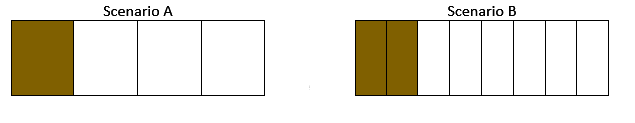### Problem 2

Find two fractions that are equivalent to each of the following:

a.     ${{1\over3}}$

b.     ${{3\over4}}$

c.     ${{3\over2}}$

### Problem 3

Use pictures to explain why the following fractions are equivalent.

a.     ${{1\over2}={3\over6}}$

b.     ${{2\over3}={8\over12}}$

c.     ${{4\over3}={12\over9}}$

## Problem Set & Homework

#### Discussion of Problem Set

• How many pieces did you shade in #3? Why?
• What answer did you choose in #4? What made these equivalent fractions different from the others we’ve looked at today?
• Look at #5c. What did your picture look like for ${{5\over2}}$? What about ${{15\over6}}$? What made these fractions different from the others?

?

### Problem 1

The model below is shaded to represent a fraction.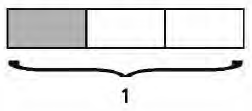Which model shows an equivalent fraction?

 A.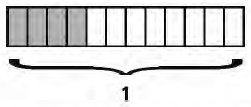B.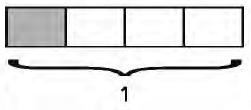C.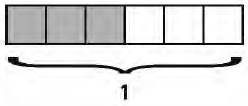D.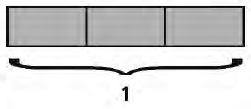#### References

Question #3

From EngageNY.org of the New York State Education Department. New York State Testing Program Grade 4 Common Core Mathematics Test Released Questions May 2016. Internet. Available from https://www.engageny.org/resource/released-2016-3-8-ela-and-mathematics-state-test-questions/file/148591; accessed March 7, 2018, 2:08 p.m..

### Problem 2

Use the pictures to explain why the two fractions represented above are equivalent.

?